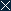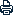Home | Sitemap | Contact | Chinese | CAS
 Search:
 About AMSS Research People International Cooperation News Societies & Journals Papers Resources Education & Training Join Us LinksLocation: Home >  Research >  Colloquia & Seminars
 （2018.4.23）Prof. Yavar Kian：Around the Calderón problem in a waveguide
 Author：
 ArticleSource：
 Update time： 2018-04-20CloseA A APrint

 Academy of Mathematics and Systems Science, CAS Colloquia & Seminars
 Speaker: Prof. Yavar Kian, Assistant Professor at the University of Aix-Marseille, France Inviter: 刘晓东 Title: Around the Calderón problem in a waveguide Time & Venue: 2018.4.23 11:00-12:00 N613 Abstract: Let $\Omega$ be an unbounded domain of $\mathbb R^3$ associated with a closed waveguide in the sense that there exists $\omega$ a bounded domain of $\mathbb R^2$ such that $\Omega\subset\omega\times\mathbb R$. In this talk, we will consider the inverse problem of determining the magnetic field associated with the magnetic potential $A\in L^\infty(\Omega)^3$ and the electric potential $q\in L^\infty(\Omega;\mathbb C)$ appearing in the magnetic Schr?inger equation $\Delta_Au+qu=0$ on $\Omega$, where $\Delta_A$ denotes the magnetic Laplacian defined by $\Delta_A= \Delta+2iA(x)\cdot\nabla +i\textrm{div}_x(A)-|A|^2$, from some data equivalent to observations of solutions on some parts of the boundary $\partial\Omega$. 报 告 人简介：Prof. Yavar Kian received his PhD degree in Applied Mathematics from the University of Bordeaux, France, in 2010. He is currently an Assistant Professor at the University of Aix-Marseille, France. He joined the University of Aix-Marseille on 2011. His current research interests include: inverse problems for different PDEs (parabolic, hyperbolic, Schr?dinger and elliptic equations), inverse spectral problems, inverse problems on manifolds, inverse and direct problems for fractional diffusion equations.
 Copyright@2008, All Rights Reserved, Academy of Mathematics and Systems Science, CAS Tel: 86-10-82541777 Fax: 86-10-82541972 E-mail: contact@amss.ac.cn